Next: D. CSP-depth migration in Up: 7: GEOMETRICAL PROBLEMS OF Previous: B. Two-layer media

## C. 15-degree algorithm in one-layer model

This example shows distortions that are connected with application of a wave-field continuation operator which is not kinematically equivalent to the classical Eiconal equation (see the next chapter).

First step: The time t0 in a true model is the same as in the example:Second step: If we apply 15-degree algorithm with the continuation velocity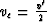, then discontinuities propagate in accordance to the Eiconal equation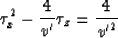(see equation (98) from Chapter 8 at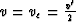).

The solution of this equation which satisfies boundary condition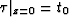is:Third step: From the conditionwe find location of the reflector image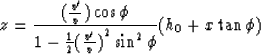whenThe factorso the depth distortion is very small for subhorizontal reflectors.Next: D. CSP-depth migration in Up: 7: GEOMETRICAL PROBLEMS OF Previous: B. Two-layer media
Stanford Exploration Project
1/13/1998